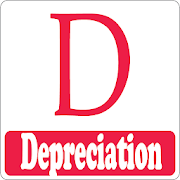# DepreciationDepreciation is an educational app for students. its a brief introduction for students.

Depreciation is defined as a reduction in the value of an asset that occurs over time as the asset gets older or as wear and tear occurs, or the decline of one currency in relation to others.

In this app include following main Topics:

*Concept of depreciation
*Objective
*Causes of depreciation
*Depreciation methods
*Depreciable assets
*Factors in measurement of depreciation
*Methods of recording depreciation
*Selection of appropriate method